# Given March 9, 2001

Problem 1. Musical Theater

A theater production of a western musical has 3 roles to fill: the villain, the sheriff, and the deputy. 15 actors try out for parts.

a) How many different possible castings are there?

This is choosing 3 people, in order, out of 15. The answer is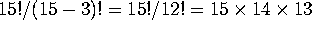. Another way to see the answer is to say there are 15 ways to cast the villain, then 14 ways to cast the sheriff, then 13 ways to cast the deputy.

b) The director decided to replace the deputy with a 3-person chorus (so there are now five parts: villain, sheriff, chorus, chorus and chorus. The three chorus parts are identical.) Again, there are 15 actors trying out for parts. How many different possible castings are there?

The answer is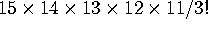, and can be obtained in several ways. One is to first cast the villain (15 ways) then the sheriff (14 ways), then the 3 chorus members (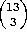ways).

Problem 2. Chess

A chess player is playing in a 10-game tournament. In each game, he has a 60% chance of winning, a 30% chance of drawing, and a 10% chance of losing, independent of how he does in the other games.

a) What is the probability of his winning exactly 5 of the 10 games?

This is binomial. In each game there is a .6 probability of winning and a .4 probability of not winning. The probability of winning 5 times out of 10 is then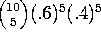.

b) What is the probability of his winning 5 games, drawing three and losing two?

This is multinomial.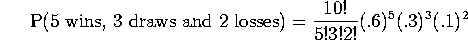c) What is the probability that he finishes the tournament with a record of 5 wins, 3 draws and 2 losses, given that he wins exactly 5 games? Please simplify your answer as much as possible.

This is the answer to (b) divided by the answer to (a). After canceling the 10!, 5!, and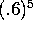, you are left with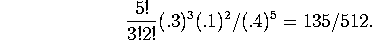Another way to understand this is to say that, each time he doesn't win, there is a (3/4) (conditional) probability of drawing and a (1/4) chance of losing. The probability that exactly 3 of the 5 non-wins are draws is then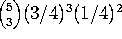.

Problem 3. Fizzbin

Fans of the old Star Trek will remember that Fizzbin is a poker-like game supposedly played on Beta Antares IV. You are dealt a 6-card hand from a standard deck. A ``Fizzbin'' is a hand with two pair plus two unmatched cards. (For example, king of spades, king of hearts, 9 of hearts, 9 of diamonds, 5 of clubs and ace of hearts). If the pairs are both 10 or higher (that is, 10, jack, queen, king or ace), then it's called a ``Royal Fizzbin''. [OK, OK, I'm making most of this up -- but so was Captain Kirk.]

a) What is the probability of being dealt a Fizzbin (either royal or regular)?

There are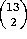ways of choosing the ranks of the two pairs, and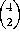ways each of choosing the suits. There are then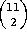ways of choosing the ranks of the two other cards, and 4 ways each of choosing the suits. There are therefore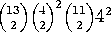different fizzbins. Since there are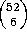possible hands, the probability of being dealt a fizzbin is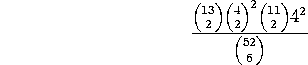b) What is the probability of being dealt a Royal Fizzbin? [When Kirk asked Spock for the odds of getting a Royal Fizzbin, Spock replied that he had never computed them. But you can!]

This is similar, except that there are only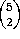ways to choose the ranks of the two pairs. So the probability of a Royal Fizzbin is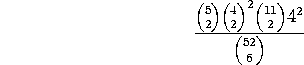Extra credit (5 points): Three jacks make a Shrok, and you get disqualified with a Shrok. What's the probability of being dealt a Shrok?

To get a Shrok you need 3 of the four jacks, and 3 of the 48 remaining cards. There are therefore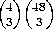possible Shroks, and the probability of a Shrok is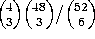.

Problem 4. Classwork

A class has 23 men and 13 women. The teacher calls on 8 (different) students to work problems at the board.

a) How many ways are there for him to pick 5 men and 3 women to do this?

There are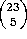ways to pick the men andways to pick the women, or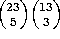ways in all.

b) If he picks the 8 students randomly, what is the probability that 5 will be men and 3 will be women?

Just divide your answer to (a) by the number of ways to choose 8 out of 36 people: P(5 men, 3 women) =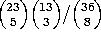. This is an example of a hypergeometric distribution.

c) One day the teacher picks the 8 students one at a time, instead of all at once. However, he is so absent-minded that he can't remember who he has already called on, so it is possible that he will select the same student(s) more than once. What is the probability that exactly 5 of the 8 students that he selects will be men?

Now the problem is binomial. Each time, there is a 23/36 chance of picking a man and a 13/36 chance of picking a woman. The probability of getting 5 men out of 8 selections is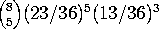.